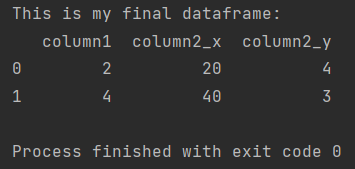Pandas How To Uncategorized How to join two dataframes on column

# How to join two dataframes on column

In this post I show how to join two dataframes on column in Pandas Python library.

You can join two pandas DataFrames on a common column by specifying the column in the on parameter of the merge method.

## Joining on column

Here’s an example of how to perform an inner join on two DataFrames based on a column named key:

```import pandas as pd

my_df1 = pd.DataFrame({'column1': [1, 2, 3, 4, 5], 'column2': [10, 20, 30, 40, 50]})
my_df2 = pd.DataFrame({'column1': [2, 4, 6, 8, 10], 'column2': [4, 3, 12, 17, 35]})

my_final_df = pd.merge(my_df1, my_df2, on='column1', how='inner')

print(f'This is my final dataframe: \n{my_final_df}')

```In this example, the on parameter is set to ‘column1’ to specify that the join should be based on that column. The how parameter is set to ‘inner’ to specify that an inner join should be performed.

The result of the join will be a new DataFrame that contains only the rows where there is a match in both input DataFrames on the chosen column. In this example, the result will have three columns: column1, column2_x, column2_y.

Tags:

## How to convert object to datetime in PandasHow to convert object to datetime in Pandas

In this post you will learn how to convert object to datetime in Pandas.

## How to turn an array into a dataframeHow to turn an array into a dataframe

You can convert a NumPy array to a Pandas DataFrame using the pd.DataFrame constructor: